## Filtering Table Calculations

by Oliver Clarke

It’s our last week of Data School, and Andy is sending us out with a bang. We’ve been going over table calculations to make sure we all have a deep and thorough understanding of them before we’re released into the world. Today we spent a bit of time talking about filtering table calculations. This is obviously a pretty useful thing to want to do, but doesn’t immediately work. In this blog, I’ll show you an example of filtering table calculations, give you the preamble explaining why it’s slightly tricky, and then give you a solution.

## Example Viz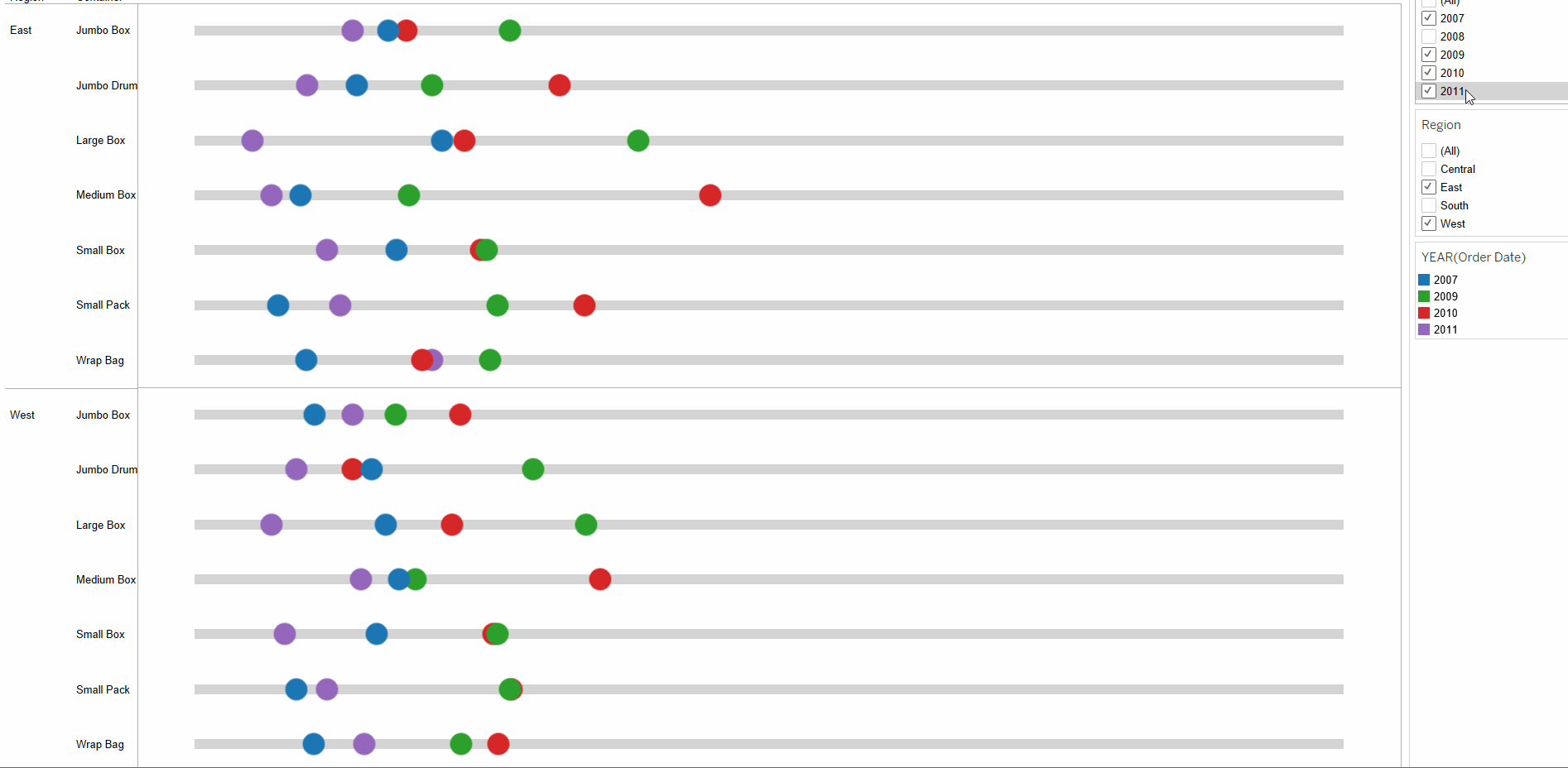So here we have a pretty simple viz. We’re looking at Regions and Containers in a slightly old version of Superstore. What we’ve plotted is the % of Total sales per container, per region that each year has contributed. As you can see, if we filter out certain years, the other years’ positions don’t move. Even though we’ve excluded 2010 for instance, the % of total doesn’t recalculate because of this exclusion. If we had just used YEAR([Order Date]) as our filter, however, then this would not be the case, and our filtering would look something like this: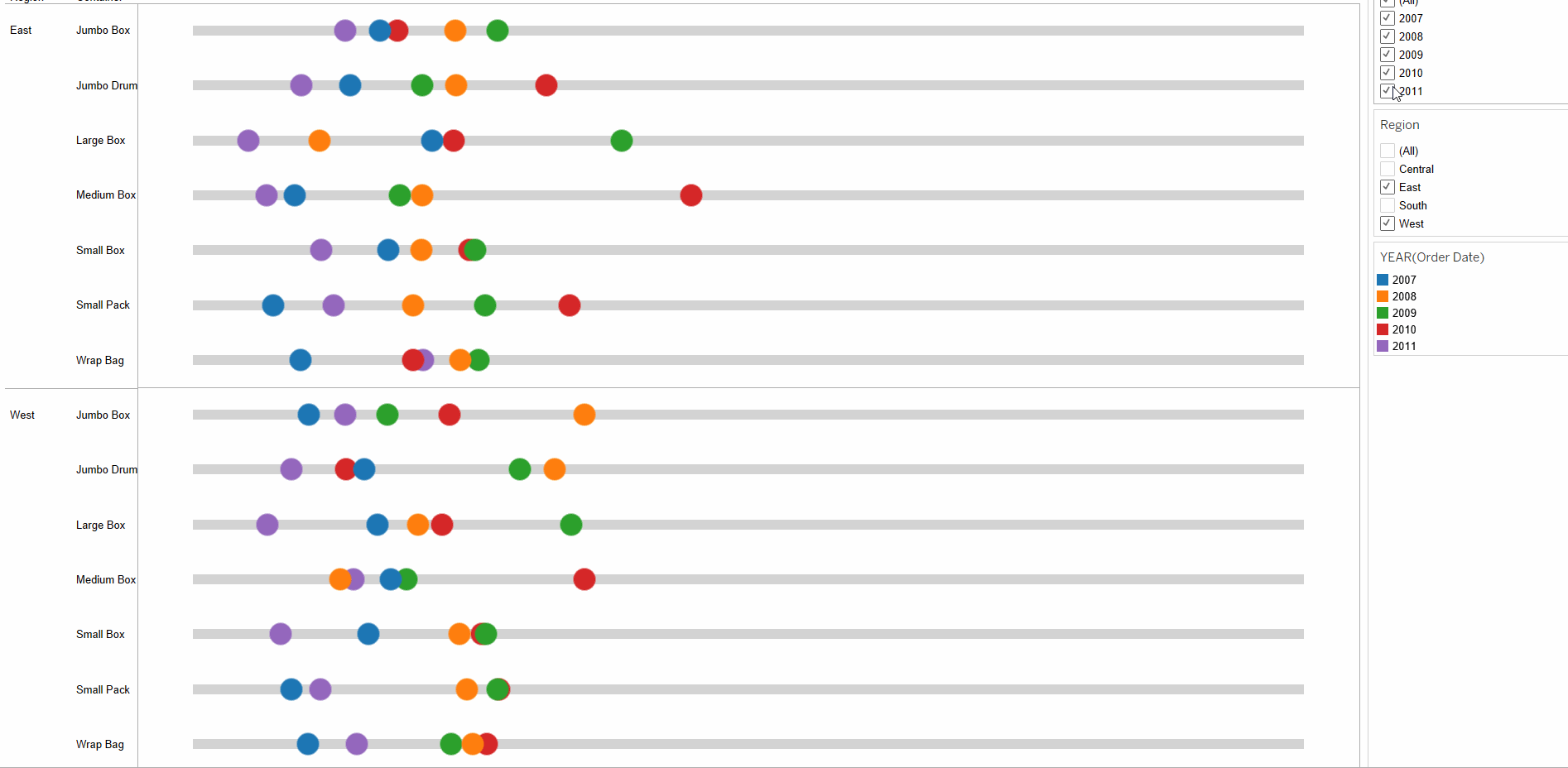Look at how the position of the dots moves

If I duplicate these sheets as crosstabs, then it might be easier to see this difference in action: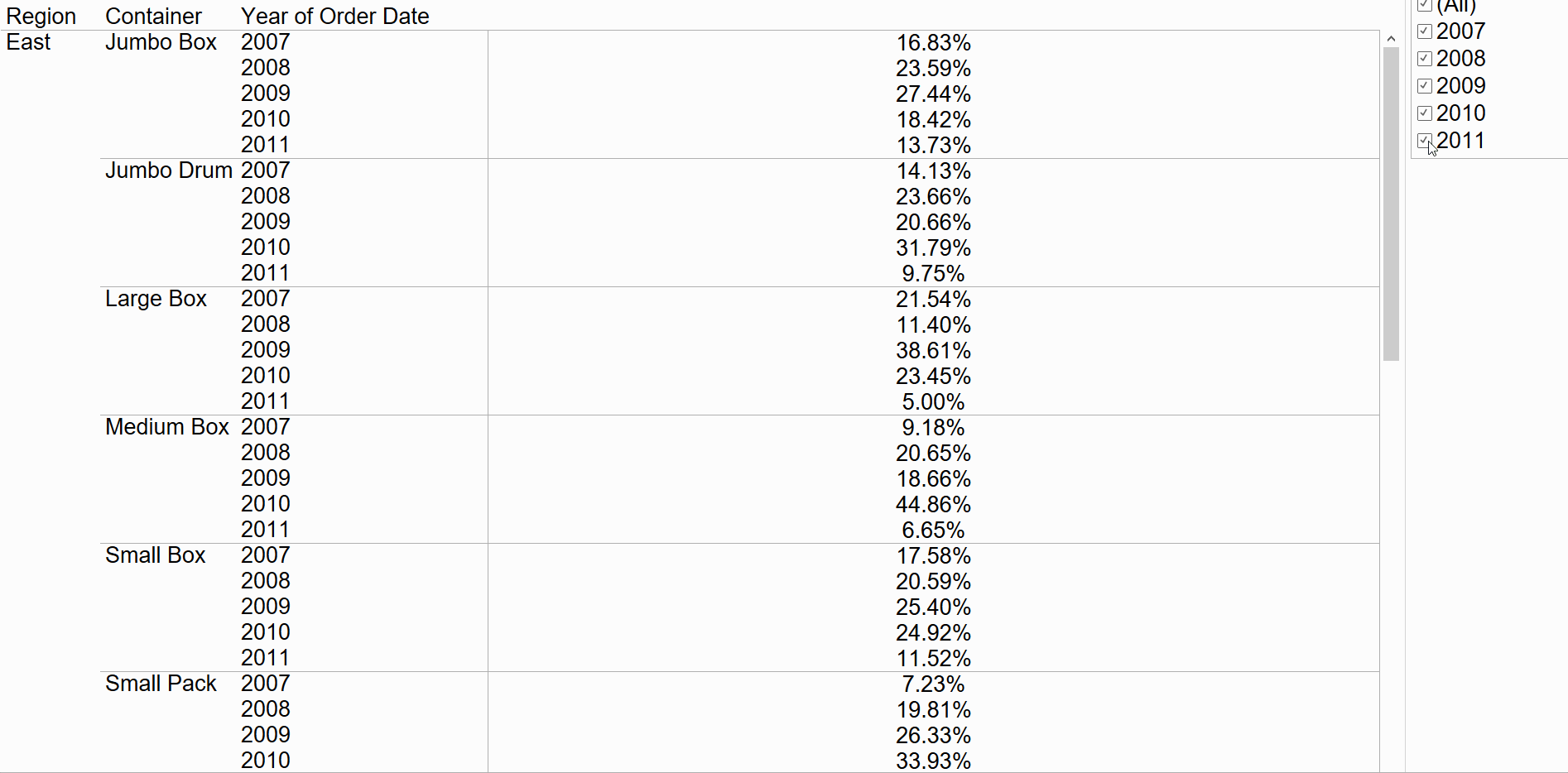With the filtered Table Calculation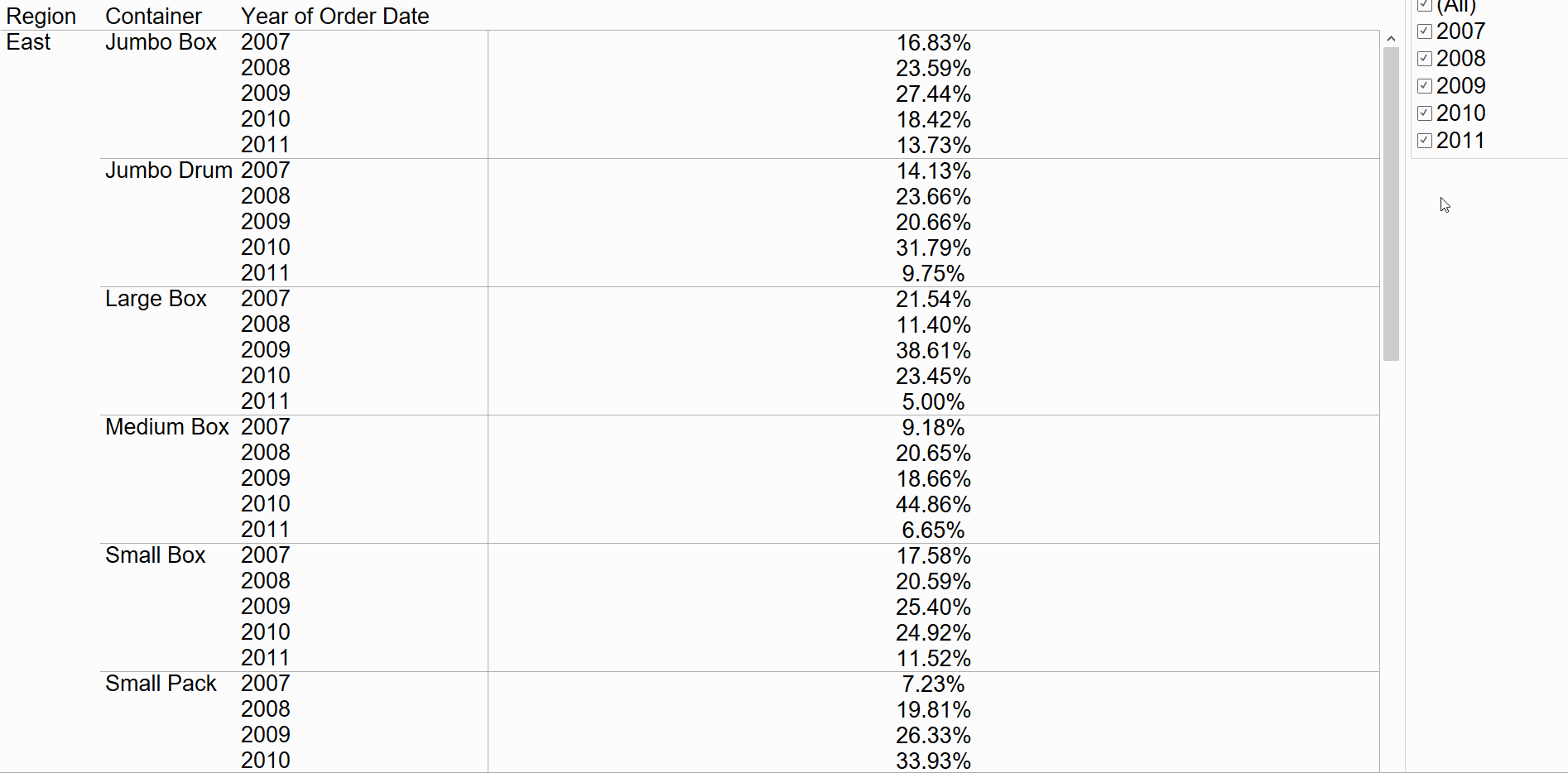With a normal filter

Using a normal dimension filter is (in this instance) screwing up our table calculations, by forcing them to recalculate when we don’t want them to. In order to explain why this is happening – and so to find a solution – we need to understand Tableau’s order of operations.

## Preamble – Order of Operations

Tableau has a clearly defined order of operations. This means that there is a strict order in which actions, or computations will happen. For the sake of this problem, we’re only interested in when dimension filters, table calculations and table calculation filters occur. The order of operations for these actions goes as follows: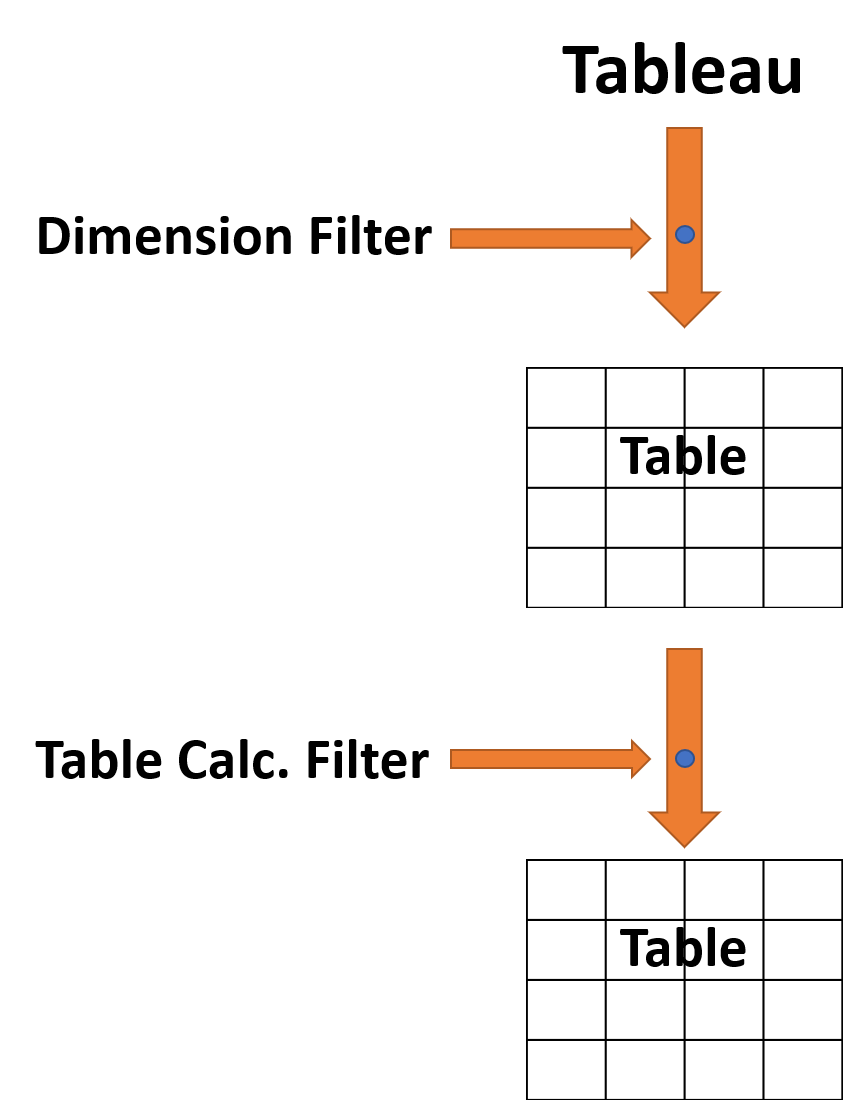Thanks to Robbin for this lovely illustration

So Tableau connects to the data (either through a live connection or to an extract). This data is aggregated into the view that we see (in the above case, a table). However, if there are any dimension filters, these are applied before the aggregated view is created. Importantly for us, since table calculations apply to what’s in the view, they, by definition, have to occur after the aggregated view has been created. Any filters which are based on table calculations occur after the table calculation stage in the order, obviously.

So earlier, when we were applying a dimension filter to our view, since that filter applies before the aggregated view (upon which table calculations are based) is created, the % of total was necessarily recalculated to reflect the changed total. If we want to stop our table calculation from recalculating, we have to base our filter on something which occurs after the aggregated view is created – i.e. a table calculation.

## Fixing the filter

We need a filter based on a table calculation which works like a normal YEAR([Order Date]) dimension filter. How are we going to get one of them? The trick, in this case, is to use the LOOKUP function with no offset. This will therefore the current value for each value, but is based on a table calculation. We use the attribute function (ATTR) as table calculations require aggregated fields. Our table calculation therefore looks like this:

LOOKUP(ATTR(YEAR([Order Date])), 0)

We then compute this using Order Date and put it on the filters shelf and we’re done 🙂

So there you have it, hopefully you understand a bit more about Tableau’s order of operations and table calculations too. If you have any questions, as always, you can find me on twitter @olliehclarke.Oliver Clarke